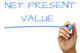# Net Present Value (NPV)

By Research Desk
about 12 months agoNet Present Value (NPV) is the difference between the amount invested on day zero i.e. today and the present value of the expected future cash receipts from today’s investment. In other words, the amount invested is compared to the future cash flow after discounting them by a specified rate of return (to make the comparison on present value terms).

Net Present Value (NPV) = Investment amount - Present value of future cash receipts

Take for Example: an investment of Rs.10 lacs made today at 10% will give Rs.11 lacs at the end of the year; therefore the present value of Rs.11 lacs at the specified rate of return (10%) is Rs.10 lacs. The amount of investment is deducted from this value of today to arrive at the net present value which in this case would be 0.

A zero NPV means the investment has repaid the original investment plus the specified rate of return. A positive NPV means a better return (over the specified rate of return) while a negative NPV means a return below the specified rate of return.